# Dynamical system problem studied by Bost--Connes

We state the problem solved by Bost and Connes in [BC] (J-B. Bost, A. Connes, Selecta Math. (New Series), 1, (1995) 411-457) and its analogue for number fields, considered in [HL](D.  Harari, E. Leichtnam, Selecta Mathematica, New Series 3 (1997), 205-243), [ALR](J. Arledge, M. Laca, I. Raeburn, Doc. Mathematica 2 (1997) 115-138) and [Coh](Paula B Cohen, J. Théorie des Nombres de Bordeaux, 11 (1999), 15-30). A (unital)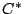-algebra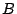is an (unital) algebra over the complex numbers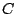with an adjoint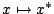,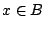, that is, an anti-linear map with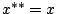,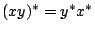,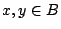, and a norm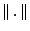with respect to whichis complete and addition and multiplication are continuous operations. One requires in addition that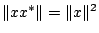for all. Adynamical system is a pair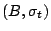, where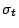is a 1-parameter group of-automorphisms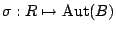. A state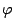on a-algebrais a positive linear functional onsatisfying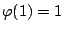. The definition of Kubo-Martin-Schwinger (KMS) of an equilibrium state at inverse temperature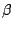is as follows.

Definition: Letbe a dynamical system, anda state on. Thenis an equilibrium state at inverse temperature, or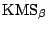-state, if for eachthere is a function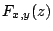, bounded and holomorphic in the band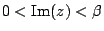and continuous on its closure, such that for all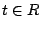,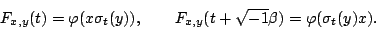(1)

A symmetry group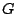of the dynamical systemis a subgroup of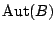commuting with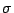: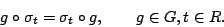Consider now a systemwith interaction. Then, guided by quantum statistical mechanics, we expect that at a critical temperature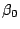a phase transition occurs and the symmetry is broken. The symmetry groupthen permutes transitively a family of extremal- states generating the possible states of the system after phase transition: the-state is no longer unique. This phase transition phenomenon is known as spontaneous symmetry breaking at the critical inverse temperature. We state the problem related to the Riemann zeta function and solved by Bost and Connes.

Problem 1: Construct a dynamical systemwith partition function the zeta function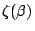of Riemann, where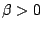is the inverse temperature, having spontaneous symmetry breaking at the pole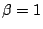of the zeta function with respect to a natural symmetry group.

The symmetry group turns out to be the unit group of the ideles, given by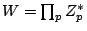where the product is over the primes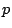and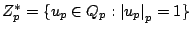. We use here the normalisation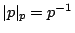. This is the same as the Galois group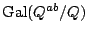, where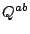is the maximal abelian extension of the rational number field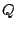. The interaction detected in the phase transition comes about from the interaction between the primes coming from considering at once all the embeddings of the non-zero rational numbers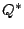into the completions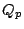ofwith respect to the prime valuations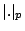. The following natural generalisation of this problem to the number field case and the Dedekind zeta function was solved in [Coh] (see also [HL], [ALR]).

Problem 2: Given a number field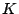, construct a dynamical systemwith partition function the Dedekind zeta function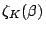, whereis the inverse temperature, having spontaneous symmetry breaking at the poleof the Dedekind function with respect to a natural symmetry group.

Back to the main index for The Riemann Hypothesis.